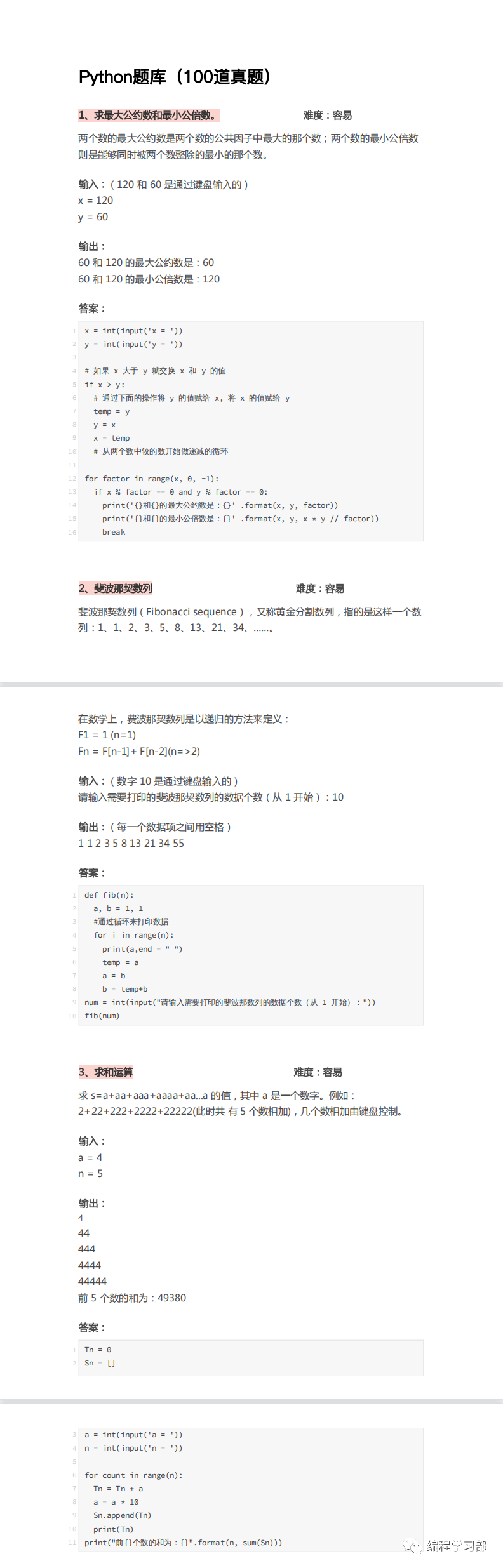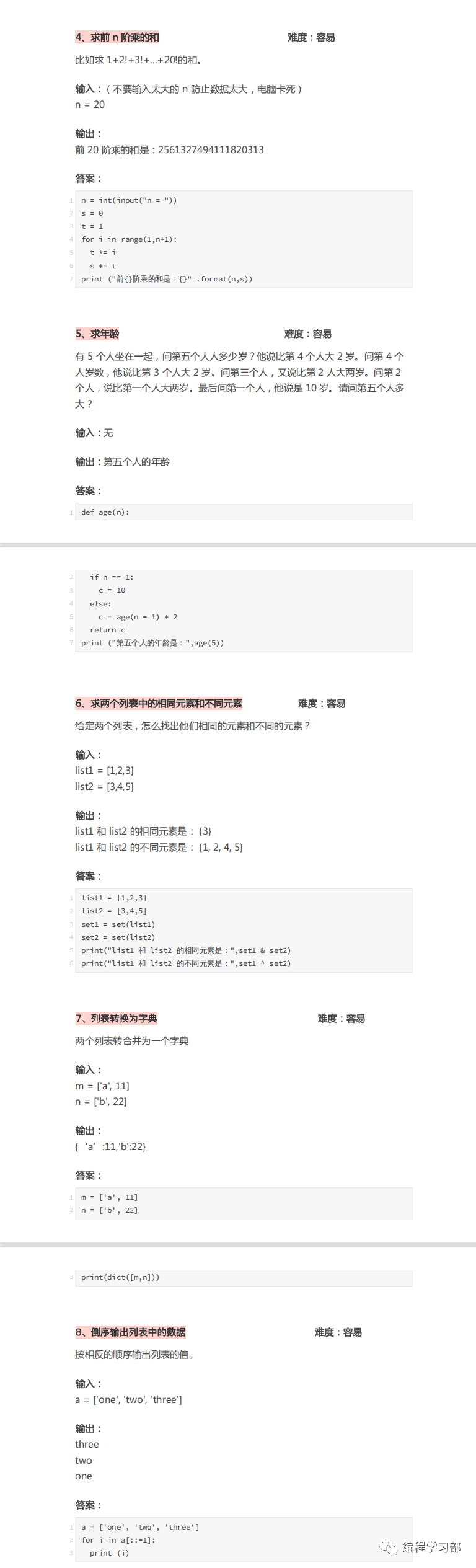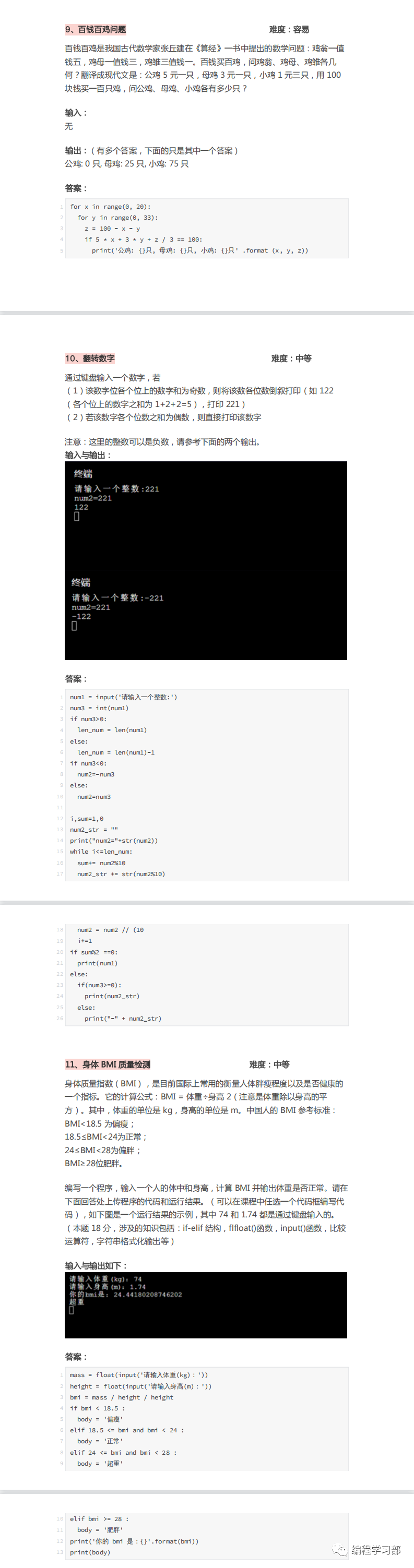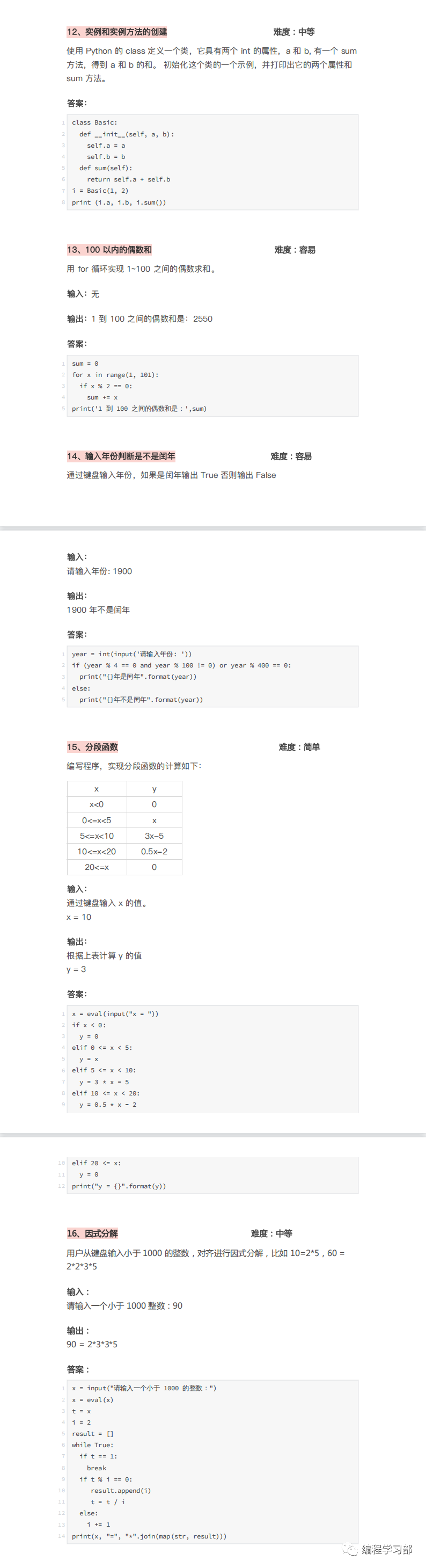### 提示：

• 使用for循环

l=[]
for i in range(2000, 3201):
if (i%7==0) and (i%5!=0):
l.append(str(i))
print ‘,’.join(l)

• 使用生成器和列表理解
`print(*(i for i in range(2000, 3201) if i%7 == 0 and i%5 != 0), sep=",")`

## 问题2

### 提示：

• 使用While循环
n = int(input()) #input() function takes input as string type
#int() converts it to integer type
fact = 1
i = 1
while i <= n:
fact = fact * i;
i = i + 1
print(fact)
• 使用For循环
n = int(input()) #input() function takes input as string type
#int() converts it to integer type
fact = 1
for i in range(1,n+1):
fact = fact * i
print(fact)
• 使用Lambda函数
n = int(input())
def shortFact(x): return 1 if x <= 1 else x*shortFact(x-1)
print(shortFact(n))

while True:
try:
num = int(input(“Enter a number: “))
break
except ValueError as err:
print(err)
org = num
fact = 1
while num:
fact = num * fact
num = num – 1
print(f’the factorial of {org} is {fact}’)

from functools import reduce
def fun(acc, item):
return acc*item
num = int(input())
print(reduce(fun,range(1, num+1), 1))

## 问题3

### 问题：

`{1: 1, 2: 4, 3: 9, 4: 16, 5: 25, 6: 36, 7: 49, 8: 64}`

### 提示：

• 使用for循环

n = int(input())
ans = {}
for i in range (1,n+1):
ans[i] = i * i
print(ans)

• 使用字典理解

n = int(input())
ans={i : i*i for i in range(1,n+1)}
print(ans)
# 演进
try:
num = int(input(“Enter a number: “))
except ValueError as err:
print(err)
dictio = dict()
for item in range(num+1):
if item == 0:
continue
else:
dictio[item] = item * item
print(dictio)

num = int(input(“Number: “))
print(dict(enumerate([i*i for i in range(1, num+1)], 1)))`0100,0011,1010,1001`

`1010`

### 提示：

def check(x): #转换二进制整数＆返回由5零中频整除
total, pw = 0, 1
reversed(x)
for i in x:
total+=pw * (ord(i) – 48) #ORD（）函数返回ASCII值
pw*=2
data = input().split(“,”) ＃输入此处，并在’，’位置分割
lst = []
for i in data:
if check(i) == 0: ＃如果零发现它是指由零整除并添加到列 lst.append(i)
print(“,”.join(lst))

def check（x）：＃如果被5整除，则check函数返回true
return int（x，2）％5 == 0 ＃int（x，b）将x作为字符串，将b作为基数，
＃将其转换为十进制

data = list（filter（check（data）））＃在filter（func，object）函数中，如果通过’check’函数
print（“，”。join（data）找到True，则从’data’中选取元素。

data = input().split(‘,’)
data = [num for num in data if int(num, 2) % 5 == 0]
print(‘,’.join(data))

## 问题12

### 提示：

lst = []
for i in range(1000,3001):
flag = 1
for j in str(i): ＃每个整数编号i被转换成字符串
if ord(j)%2 != 0: ＃ORD返回ASCII值并且j是i
flag = 0
if flag == 1:
lst.append(str(i)) ＃i作为字符串存储在列表中
print(“,”.join(lst))

def check(element):
return all(ord(i)%2 == 0 for i in element) ＃所有返回true如果所有的数字，i是即使在元件
lst = [str(i) for i in range(1000,3001)] ＃创建所有给定数字的列表，其字符串数据类型为
lst = list(filter(check,lst)) ＃如果检查条件失败，则过滤器从列表中删除元素
print(“,”.join(lst))
lst = [str(i) for i in range(1000,3001)]
lst = list(filter(lambda i:all(ord(j)%2 == 0 for j in i), lst)) ＃使用lambda来在过滤器功能内部定义函数
print(“,”.join(lst))

## 问题13

### 问题：

`hello world! 123`

LETTERS 10
DIGITS 3

### 提示：

word = input()
letter,digit = 0,0
for i in word:
if (‘a'<=i and i<=’z’) or (‘A'<=i and i<=’Z’):
letter+=1
if ‘0’<=i and i<=’9′:
digit+=1
print(“LETTERS {0}\nDIGITS {1}”.format(letter,digit))

word = input()
letter, digit = 0,0
for i in word:
if i.isalpha(): ＃返回true如果字母表
letter += 1
elif i.isnumeric(): ＃返回true如果数字
digit += 1
print(f”LETTERS {letter}\n{digits}”) ＃两种解决方案均显示两种不同类型的格式化方法

10-13以上所有问题大多是与字符串有关的问题。解决方案的主要部分包括字符串替换函数和理解方法，以更短的形式写下代码。

## 问题14

### 问题：

`Hello world!`

UPPER CASE 1
LOWER CASE 9

### 提示：

word = input()
upper,lower = 0,0
for i in word:
if ‘a'<=i and i<=’z’ :
lower+=1
if ‘A'<=i and i<=’Z’:
upper+=1
print(“UPPER CASE {0}\nLOWER CASE {1}”.format(upper,lower))

word = input()
upper,lower = 0,0
for i in word:
lower+=i.islower()
upper+=i.isupper()
print(“UPPER CASE {0}\nLOWER CASE {1}”.format(upper,lower))

string = input(“Enter the sentense”)
upper = 0
lower = 0
for x in string:
if x.isupper() == True:
upper += 1
if x.islower() == True:
lower += 1
print(“UPPER CASE: “, upper)
print(“LOWER CASE: “, lower)